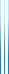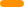# PublicationsPapers

## Average case lower bounds for monotone switching networks

Yuval Filmus, Toniann Pitassi, Robert Robere and Stephen A. Cook
FOCS 2013

An approximate computation of a Boolean function f by a circuit or switching network M is a computation in which M computes f correctly on the majority of the inputs (rather than on all of them). Besides being interesting in their own right, lower bounds for approximate computation have proved useful in many subareas of complexity theory such as cryptography and derandomization. Lower bounds for approximate computation are also known as correlation bounds or average case hardness.

We obtain the first average case monotone depth lower bounds for a function in monotone $\mathsf{P}$. We tolerate errors up to $1/2 – 1/n^{1/3-\delta}$. Specifically, we prove average case exponential lower bounds on the size of monotone switching networks for the GEN function. As a corollary, we establish that for every $i$ there are functions that can be computed with no error in monotone $\mathsf{NC}^{i+1}$ but that cannot be computed without large error by monotone circuits in $\mathsf{NC}^i$. We provide a similar separation between monotone $\mathsf{NC}$ and monotone $\mathsf{P}$.

Our proof extends and simplifies the Fourier-analytic technique due to Potechin and further developed by Chan and Potechin.

As a corollary of our main lower bound, we prove that the communication complexity approach for monotone depth lower bounds does not naturally generalize to the average case setting.

## BibTeX

@inproceedings{FPRC2013,
author = {Yuval Filmus and Toniann Pitassi and Robert Robere and
Stephen A. Cook},
title = {Average case lower bounds for monotone switching networks},
booktitle = {The 54th Annual Symposium on Foundations of Computer
Science ({FOCS} 2013)},
pages = {598--607},
year = {2013}
}copy to clipboard